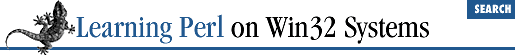home | O'Reilly's CD bookshelfs | FreeBSD | Linux | Cisco | Cisco Exam

### 11.2 Defining a Format

A format is defined using a format definition. This format definition can appear anywhere in your program text, like a subroutine. A format definition looks like this:

```format someformatname =
fieldline
value_one, value_two, value_three
fieldline
value_one, value_two
fieldline
value_one, value_two, value_three
.```

The first line contains the reserved word ``` format``` , followed by the format name and then an equal sign (``` =``` ). The format name is chosen from yet another namespace, and follows the same rule as everything else. Because format names are never used within the body of the program (except within string values), you can safely use names that are identical to reserved words. As you'll see in the following section, "Invoking a Format ," most of your format names will probably be the same as filehandle names (which then makes them not the same as reserved words...oh well).

Following the first line comes the template itself, spanning zero or more text lines. The end of the template is indicated by a line consisting of a single dot by itself.[ 2 ] Templates are sensitive to whitespace; this instance is one of the few in which the kind and amount of whitespace (space, newline, or tab) in the text of a Perl program actually matters.

 In text files, the last line needs to end with a newline to work properly.

The template definition contains a series of fieldlines . Each fieldline may contain fixed text - text that will be printed out literally when the format is invoked. Here's an example of a fieldline with fixed text:

`Hello, my name is Fred Flintstone.`

Fieldlines may also contain fieldholders for variable text. If a line contains fieldholders, the following line of the template (called the value line) dictates a series of scalar values - one per fieldholder - that provide the values that will be plugged into the fields. Here's an example of a fieldline with one fieldholder and the value line that follows:

```Hello, my name is @<<<<<<<<<<
\$name```

The fieldholder is the ``` @<<<<<<<<<<``` , which specifies a left-justified text field with 11 characters. More complete details about fieldholders will be given in the section called "More About the Fieldholders " later in this chapter.

If the fieldline has multiple fieldholders, it needs multiple values, so the values are separated on the value line by commas:

```Hello, my name is @<<<<<<<<<< and I'm @<< years old.
\$name, \$age```

Putting all this together, we can create a simple format for an address label:

```format ADDRESSLABEL =
===============================
| @<<<<<<<<<<<<<<<<<<<<<<<<<< |
\$name
| @<<<<<<<<<<<<<<<<<<<<<<<<<< |
| @<<<<<<<<<<<<<<<<, @< @<<<< |
\$city,          \$state, \$zip
===============================
.```

Note that the lines of equal signs at the top and bottom of the format have no fields, and thus have no value lines following. (If you put a value line following such a fieldline, it will be interpreted as another fieldline, and will most likely not do what you want.)

Whitespace within the value line is ignored. Some people choose to use additional whitespace in the value line to line up the variable with the fieldholder on the preceding line (such as putting ``` \$zip``` underneath the third field of the previous line in this example), but that's just for looks. Perl doesn't care, and the change doesn't affect your output.

Text after the first newline in a value is discarded (except in the special case of multiline fieldholders, described later).

A format definition is like a subroutine definition. It doesn't contain immediately executed code, and can therefore be placed anywhere in the file with the rest of the program - we tend to put ours toward the end of the file, ahead of my subroutine definitions.11.1 What Is a Format?11.3 Invoking a Format